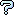All about flooble | fun stuff | Get a free chatterbox | Free JavaScript | Avatarsperplexus dot infoDivisibility of N (Posted on 2013-06-15)The problem Divisibility of 29 essentially asks whether three 4th powers can sum to a multiple of 29 if they are not all multiples of 29.

The entry for 29 in The Penguin Dictionary of Curious and Interesting Numbers by David Wells (1987) contains:

No sum of three 4th powers is divisible by either 5 or 29 unless they all are. [Euler]

1. If three aren't enough, how many 4th powers does it take to be divisible by either 5 or 29 where they aren't all?

2. If possible, find the next number beyond 5 and 29 that does not divide a sum of three 4th powers.

3. Prove every even number takes at most two 4th powers.
For example using 18 we have 34+154 = 50706 = 18*2817

4. What is the largest number of 5th powers whose sum is divisible by a number N where they aren't all divisible by N?

5. Prove that for higher powers, there is no limit to how many numbers it can take.

 No Solution Yet Submitted by Jer No RatingComments: ( Back to comment list | You must be logged in to post comments.)No Subject| Comment 1 of 4
Prove every even number takes at most two 4th powers.

WHAT DOES IT MEAN??
every even number ??
2, 4,    100?

please explain:  for every  even divisor 2k there exist  a number 2mk , equal to a^2+b^2, none of those addends divisible by 2k .

Is this the thing to be proven??

Edited on June 15, 2013, 2:38 pm
 Posted by Ady TZIDON on 2013-06-15 14:14:25Please log in:

 Search: Search body:
Forums (0)